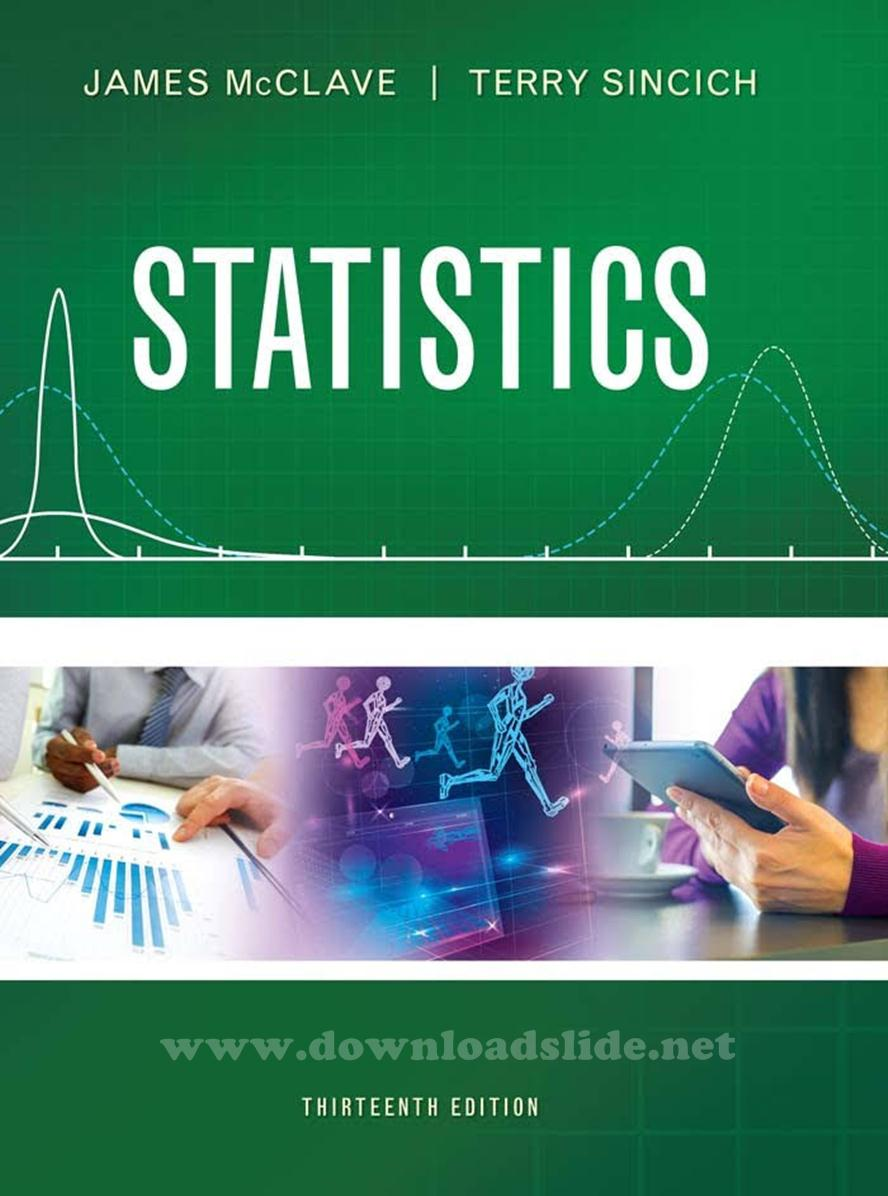### Ebook Statistics 13e by McClave, SincichStatistics
13th Edition
by James T. McClave - Terry T. Sincich
©2017 | Pe*rson Edu*ation / Pr*ntice Hall
PDF

Table of Content:
1. Statistics, Data, and Statistical Thinking
2. Methods for Describing Sets of Data
3. Probability
4. Discrete Random Variables
5. Continuous Random Variables
6. Sampling Distributions
7. Inferences Based on a Single Sample: Estimation with Confidence Intervals
8. Inferences Based on a Single
9. Inferences Based on Two Samples: Confidence Intervals and Tests of Hypotheses
10. Analysis of Variance: Comparing More than Two Means
11. Simple Linear Regression
12. Multiple Regression and Model Building
13. Categorical Data Analysis
14. Nonparametric Statistics (available online)